# Time Series Analysis in Python – A Comprehensive Guide with Examples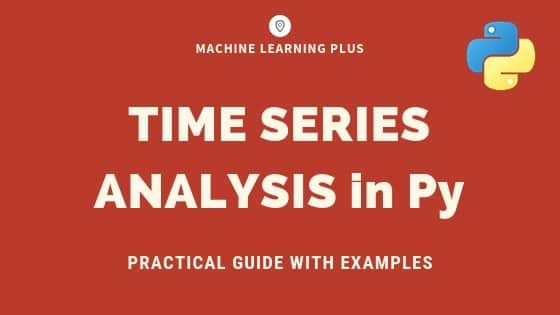Time series is a sequence of observations recorded at regular time intervals. This guide walks you through the process of analyzing the characteristics of a given time series in python.Time Series Analysis in Python – A Comprehensive Guide. Photo by Daniel Ferrandiz.

## Contents

1. What is a Time Series?
2. How to import Time Series in Python?
3. What is panel data?
4. Visualizing a Time Series
5. Patterns in a Time Series
6. Additive and multiplicative Time Series
7. How to decompose a Time Series into its components?
8. Stationary and non-stationary Time Series
9. How to make a Time Series stationary?
10. How to test for stationarity?
11. What is the difference between white noise and a stationary series?
12. How to detrend a Time Series?
13. How to deseasonalize a Time Series?
14. How to test for seasonality of a Time Series?
15. How to treat missing values in a Time Series?
16. What is autocorrelation and partial autocorrelation functions?
17. How to compute partial autocorrelation function?
18. Lag Plots
19. How to estimate the forecastability of a Time Series?
20. Why and How to smoothen a Time Series?
21. How to use Granger Causality test to know if one Time Series is helpful in forecasting another?
22. What Next

## 1. What is a Time Series?

Time series is a sequence of observations recorded at regular time intervals.

Depending on the frequency of observations, a time series may typically be hourly, daily, weekly, monthly, quarterly and annual. Sometimes, you might have seconds and minute-wise time series as well, like, number of clicks and user visits every minute etc.

Why even analyze a time series?

Because it is the preparatory step before you develop a forecast of the series.

Besides, time series forecasting has enormous commercial significance because stuff that is important to a business like demand and sales, number of visitors to a website, stock price etc are essentially time series data.

So what does analyzing a time series involve?

Time series analysis involves understanding various aspects about the inherent nature of the series so that you are better informed to create meaningful and accurate forecasts.

## 2. How to import time series in python?

So how to import time series data?

The data for a time series typically stores in .csv files or other spreadsheet formats and contains two columns: the date and the measured value.

Let’s use the read_csv() in pandas package to read the time series dataset (a csv file on Australian Drug Sales) as a pandas dataframe. Adding the parse_dates=['date'] argument will make the date column to be parsed as a date field.

from dateutil.parser import parse
import matplotlib as mpl
import matplotlib.pyplot as plt
import seaborn as sns
import numpy as np
import pandas as pd
plt.rcParams.update({'figure.figsize': (10, 7), 'figure.dpi': 120})

# Import as Dataframe
df = pd.read_csv('https://raw.githubusercontent.com/selva86/datasets/master/a10.csv', parse_dates=['date'])
df.head()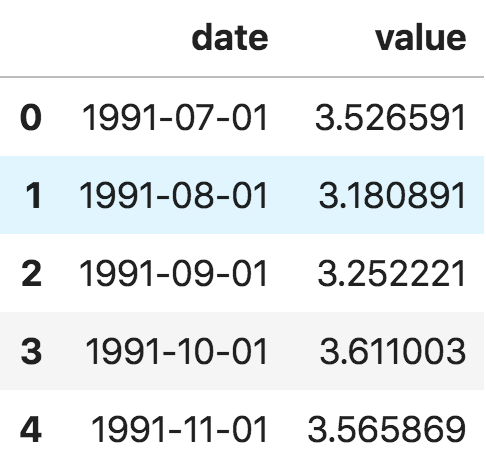Dataframe Time Series

Alternately, you can import it as a pandas Series with the date as index. You just need to specify the index_col argument in the pd.read_csv() to do this.

ser = pd.read_csv('https://raw.githubusercontent.com/selva86/datasets/master/a10.csv', parse_dates=['date'], index_col='date')
ser.head()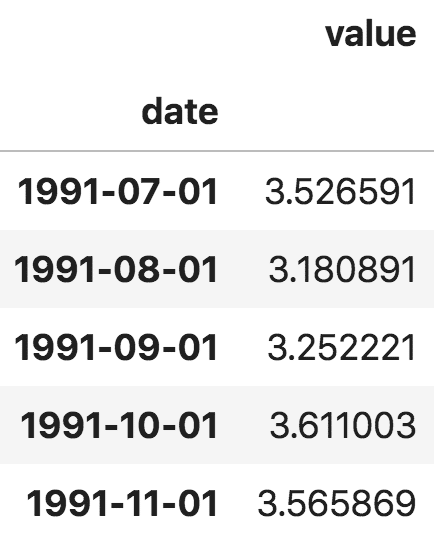Series Timeseries

Note, in the series, the ‘value’ column is placed higher than date to imply that it is a series.

## 3. What is panel data?

Panel data is also a time based dataset.

The difference is that, in addition to time series, it also contains one or more related variables that are measured for the same time periods.

Typically, the columns present in panel data contain explanatory variables that can be helpful in predicting the Y, provided those columns will be available at the future forecasting period.

An example of panel data is shown below.

# dataset source: https://github.com/rouseguy
df = pd.read_csv('https://raw.githubusercontent.com/selva86/datasets/master/MarketArrivals.csv')
df = df.loc[df.market=='MUMBAI', :]
df.head()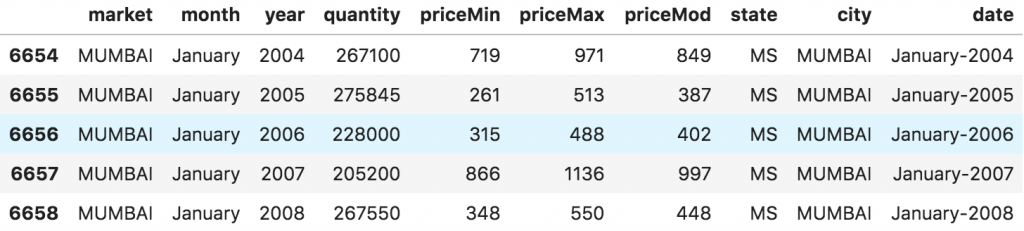Panel Data

## 4. Visualizing a time series

Let’s use matplotlib to visualise the series.

# Time series data source: fpp pacakge in R.
import matplotlib.pyplot as plt
df = pd.read_csv('https://raw.githubusercontent.com/selva86/datasets/master/a10.csv', parse_dates=['date'], index_col='date')

# Draw Plot
def plot_df(df, x, y, title="", xlabel='Date', ylabel='Value', dpi=100):
plt.figure(figsize=(16,5), dpi=dpi)
plt.plot(x, y, color='tab:red')
plt.gca().set(title=title, xlabel=xlabel, ylabel=ylabel)
plt.show()

plot_df(df, x=df.index, y=df.value, title='Monthly anti-diabetic drug sales in Australia from 1992 to 2008.')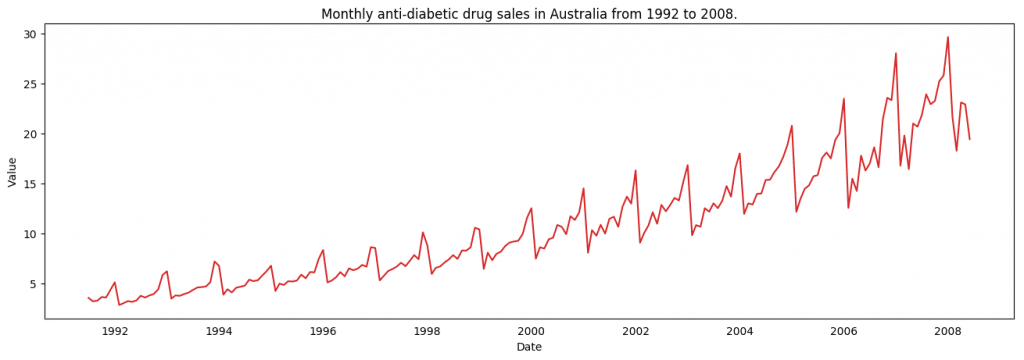Visualizing Time Series

Since all values are positive, you can show this on both sides of the Y axis to emphasize the growth.

# Import data
df = pd.read_csv('datasets/AirPassengers.csv', parse_dates=['date'])
x = df['date'].values
y1 = df['value'].values

# Plot
fig, ax = plt.subplots(1, 1, figsize=(16,5), dpi= 120)
plt.fill_between(x, y1=y1, y2=-y1, alpha=0.5, linewidth=2, color='seagreen')
plt.ylim(-800, 800)
plt.title('Air Passengers (Two Side View)', fontsize=16)
plt.hlines(y=0, xmin=np.min(df.date), xmax=np.max(df.date), linewidth=.5)
plt.show()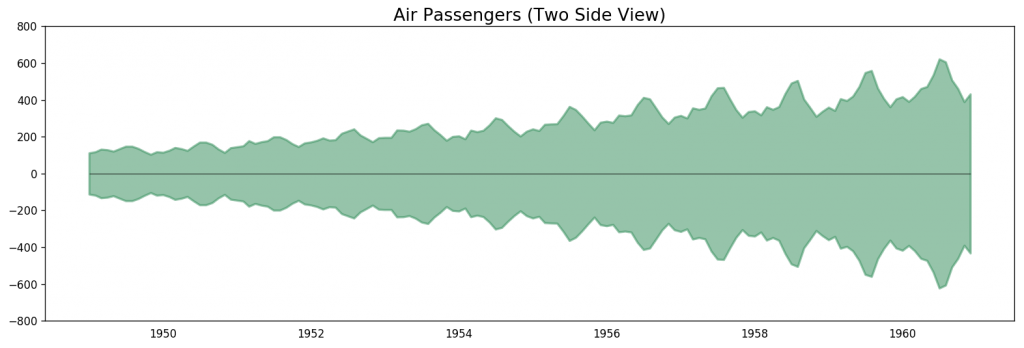Air Passengers Data – 2 Side Series

Since its a monthly time series and follows a certain repetitive pattern every year, you can plot each year as a separate line in the same plot. This lets you compare the year wise patterns side-by-side.

Seasonal Plot of a Time Series

# Import Data
df = pd.read_csv('https://raw.githubusercontent.com/selva86/datasets/master/a10.csv', parse_dates=['date'], index_col='date')
df.reset_index(inplace=True)

# Prepare data
df['year'] = [d.year for d in df.date]
df['month'] = [d.strftime('%b') for d in df.date]
years = df['year'].unique()

# Prep Colors
np.random.seed(100)
mycolors = np.random.choice(list(mpl.colors.XKCD_COLORS.keys()), len(years), replace=False)

# Draw Plot
plt.figure(figsize=(16,12), dpi= 80)
for i, y in enumerate(years):
if i > 0:
plt.plot('month', 'value', data=df.loc[df.year==y, :], color=mycolors[i], label=y)
plt.text(df.loc[df.year==y, :].shape-.9, df.loc[df.year==y, 'value'][-1:].values, y, fontsize=12, color=mycolors[i])

# Decoration
plt.gca().set(xlim=(-0.3, 11), ylim=(2, 30), ylabel='$Drug Sales$', xlabel='$Month$')
plt.yticks(fontsize=12, alpha=.7)
plt.title("Seasonal Plot of Drug Sales Time Series", fontsize=20)
plt.show()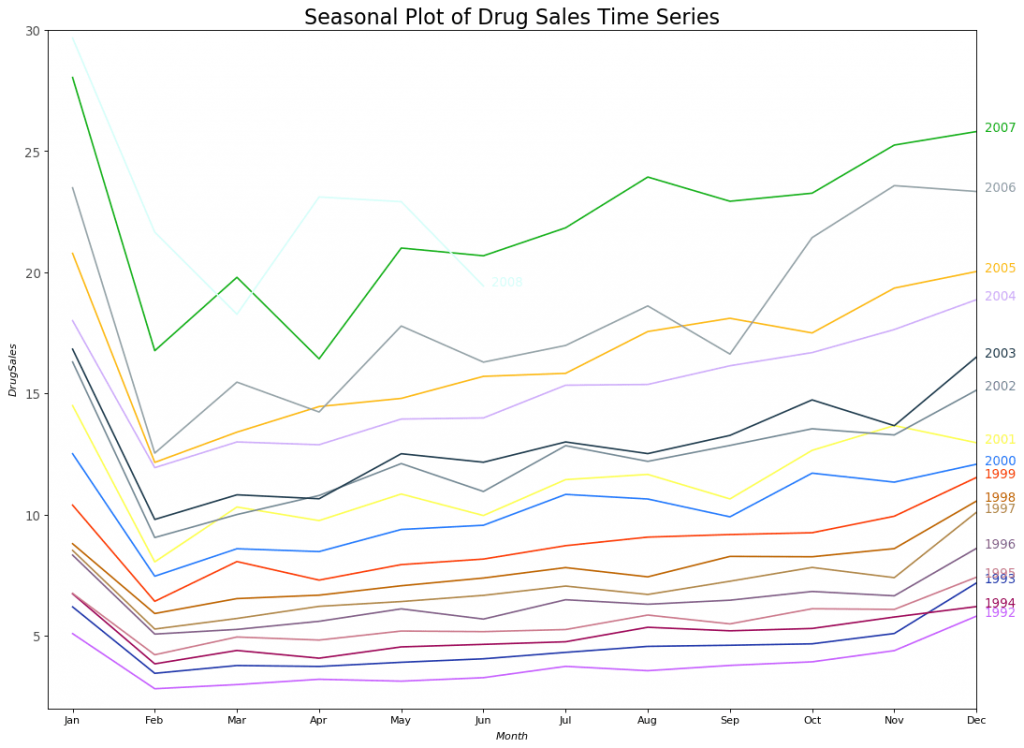Seasonal Plot of Drug Sales

There is a steep fall in drug sales every February, rising again in March, falling again in April and so on. Clearly, the pattern repeats within a given year, every year.

However, as years progress, the drug sales increase overall. You can nicely visualize this trend and how it varies each year in a nice year-wise boxplot. Likewise, you can do a month-wise boxplot to visualize the monthly distributions.

Boxplot of Month-wise (Seasonal) and Year-wise (trend) Distribution

You can group the data at seasonal intervals and see how the values are distributed within a given year or month and how it compares over time.

# Import Data
df = pd.read_csv('https://raw.githubusercontent.com/selva86/datasets/master/a10.csv', parse_dates=['date'], index_col='date')
df.reset_index(inplace=True)

# Prepare data
df['year'] = [d.year for d in df.date]
df['month'] = [d.strftime('%b') for d in df.date]
years = df['year'].unique()

# Draw Plot
fig, axes = plt.subplots(1, 2, figsize=(20,7), dpi= 80)
sns.boxplot(x='year', y='value', data=df, ax=axes)
sns.boxplot(x='month', y='value', data=df.loc[~df.year.isin([1991, 2008]), :])

# Set Title
axes.set_title('Year-wise Box Plot\n(The Trend)', fontsize=18);
axes.set_title('Month-wise Box Plot\n(The Seasonality)', fontsize=18)
plt.show()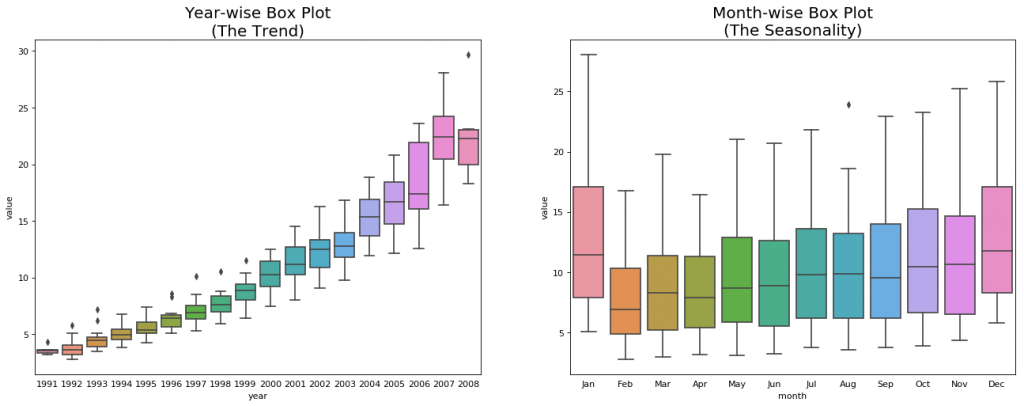Yearwise and Monthwise Boxplot

The boxplots make the year-wise and month-wise distributions evident. Also, in a month-wise boxplot, the months of December and January clearly has higher drug sales, which can be attributed to the holiday discounts season.

So far, we have seen the similarities to identify the pattern. Now, how to find out any deviations from the usual pattern?

## 5. Patterns in a time series

Any time series may be split into the following components: Base Level + Trend + Seasonality + Error

A trend is observed when there is an increasing or decreasing slope observed in the time series. Whereas seasonality is observed when there is a distinct repeated pattern observed between regular intervals due to seasonal factors. It could be because of the month of the year, the day of the month, weekdays or even time of the day.

However, It is not mandatory that all time series must have a trend and/or seasonality. A time series may not have a distinct trend but have a seasonality. The opposite can also be true.

So, a time series may be imagined as a combination of the trend, seasonality and the error terms.

fig, axes = plt.subplots(1,3, figsize=(20,4), dpi=100)
pd.read_csv('https://raw.githubusercontent.com/selva86/datasets/master/guinearice.csv', parse_dates=['date'], index_col='date').plot(title='Trend Only', legend=False, ax=axes)

pd.read_csv('https://raw.githubusercontent.com/selva86/datasets/master/sunspotarea.csv', parse_dates=['date'], index_col='date').plot(title='Seasonality Only', legend=False, ax=axes)

pd.read_csv('https://raw.githubusercontent.com/selva86/datasets/master/AirPassengers.csv', parse_dates=['date'], index_col='date').plot(title='Trend and Seasonality', legend=False, ax=axes)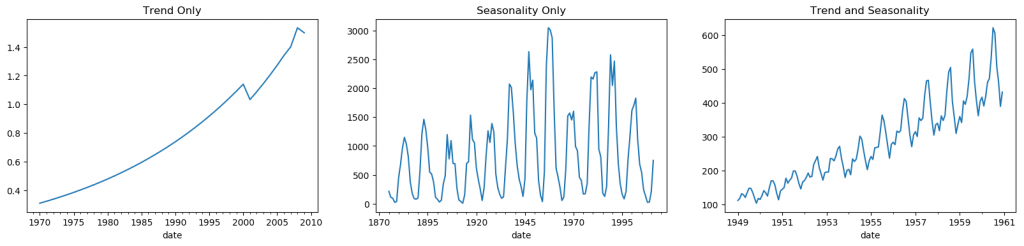Patterns in Time Series

Another aspect to consider is the cyclic behaviour. It happens when the rise and fall pattern in the series does not happen in fixed calendar-based intervals. Care should be taken to not confuse ‘cyclic’ effect with ‘seasonal’ effect.

So, How to diffentiate between a ‘cyclic’ vs ‘seasonal’ pattern?

If the patterns are not of fixed calendar based frequencies, then it is cyclic. Because, unlike the seasonality, cyclic effects are typically influenced by the business and other socio-economic factors.

## 6. Additive and multiplicative time series

Depending on the nature of the trend and seasonality, a time series can be modeled as an additive or multiplicative, wherein, each observation in the series can be expressed as either a sum or a product of the components:

Additive time series:
Value = Base Level + Trend + Seasonality + Error

Multiplicative Time Series:
Value = Base Level x Trend x Seasonality x Error

## 7. How to decompose a time series into its components?

You can do a classical decomposition of a time series by considering the series as an additive or multiplicative combination of the base level, trend, seasonal index and the residual.

The seasonal_decompose in statsmodels implements this conveniently.

from statsmodels.tsa.seasonal import seasonal_decompose
from dateutil.parser import parse

# Import Data
df = pd.read_csv('https://raw.githubusercontent.com/selva86/datasets/master/a10.csv', parse_dates=['date'], index_col='date')

# Multiplicative Decomposition
result_mul = seasonal_decompose(df['value'], model='multiplicative', extrapolate_trend='freq')

# Additive Decomposition
result_add = seasonal_decompose(df['value'], model='additive', extrapolate_trend='freq')

# Plot
plt.rcParams.update({'figure.figsize': (10,10)})
result_mul.plot().suptitle('Multiplicative Decompose', fontsize=22)
result_add.plot().suptitle('Additive Decompose', fontsize=22)
plt.show()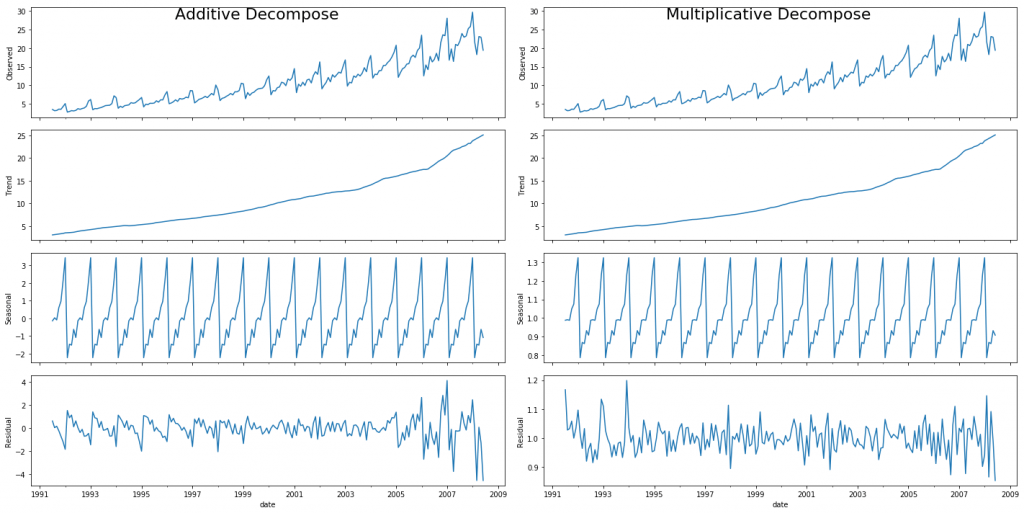Additive and Multiplicative Decompose

Setting extrapolate_trend='freq' takes care of any missing values in the trend and residuals at the beginning of the series.

If you look at the residuals of the additive decomposition closely, it has some pattern left over. The multiplicative decomposition, however, looks quite random which is good. So ideally, multiplicative decomposition should be preferred for this particular series.

The numerical output of the trend, seasonal and residual components are stored in the result_mul output itself. Let’s extract them and put it in a dataframe.

# Extract the Components ----
# Actual Values = Product of (Seasonal * Trend * Resid)
df_reconstructed = pd.concat([result_mul.seasonal, result_mul.trend, result_mul.resid, result_mul.observed], axis=1)
df_reconstructed.columns = ['seas', 'trend', 'resid', 'actual_values']
df_reconstructed.head()


If you check, the product of seas, trend and resid columns should exactly equal to the actual_values.

## 8. Stationary and Non-Stationary Time Series

Stationarity is a property of a time series. A stationary series is one where the values of the series is not a function of time.

That is, the statistical properties of the series like mean, variance and autocorrelation are constant over time. Autocorrelation of the series is nothing but the correlation of the series with its previous values, more on this coming up.

A stationary time series id devoid of seasonal effects as well.

So how to identify if a series is stationary or not? Let’s plot some examples to make it clear: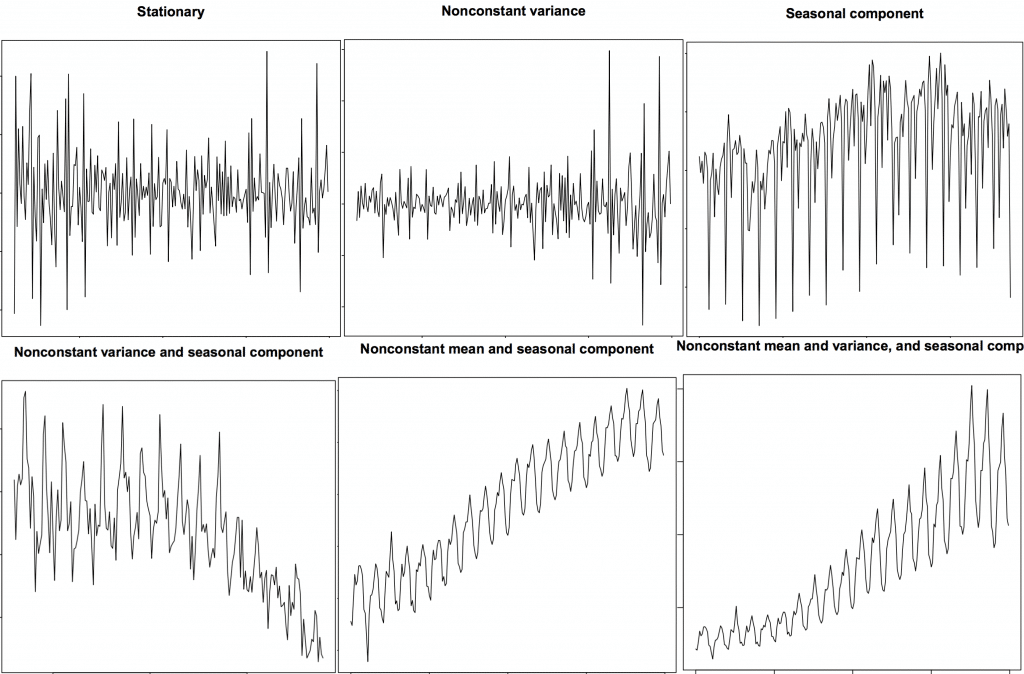Stationary and Non-Stationary Time Series

The above image is sourced from R’s TSTutorial.

So why does a stationary series matter? why am I even talking about it?

I will come to that in a bit, but understand that it is possible to make nearly any time series stationary by applying a suitable transformation. Most statistical forecasting methods are designed to work on a stationary time series. The first step in the forecasting process is typically to do some transformation to convert a non-stationary series to stationary.

## 9. How to make a time series stationary?

You can make series stationary by:

1. Differencing the Series (once or more)
2. Take the log of the series
3. Take the nth root of the series
4. Combination of the above

The most common and convenient method to stationarize the series is by differencing the series at least once until it becomes approximately stationary.

So what is differencing?

If Y_t is the value at time ‘t’, then the first difference of Y = Yt – Yt-1. In simpler terms, differencing the series is nothing but subtracting the next value by the current value.

If the first difference doesn’t make a series stationary, you can go for the second differencing. And so on.

For example, consider the following series: [1, 5, 2, 12, 20]

First differencing gives: [5-1, 2-5, 12-2, 20-12] = [4, -3, 10, 8]

Second differencing gives: [-3-4, -10-3, 8-10] = [-7, -13, -2]

## 9. Why make a non-stationary series stationary before forecasting?

Forecasting a stationary series is relatively easy and the forecasts are more reliable.

An important reason is, autoregressive forecasting models are essentially linear regression models that utilize the lag(s) of the series itself as predictors.

We know that linear regression works best if the predictors (X variables) are not correlated against each other. So, stationarizing the series solves this problem since it removes any persistent autocorrelation, thereby making the predictors(lags of the series) in the forecasting models nearly independent.

Now that we’ve established that stationarizing the series important, how do you check if a given series is stationary or not?

## 10. How to test for stationarity?

The stationarity of a series can be established by looking at the plot of the series like we did earlier.

Another method is to split the series into 2 or more contiguous parts and computing the summary statistics like the mean, variance and the autocorrelation. If the stats are quite different, then the series is not likely to be stationary.

Nevertheless, you need a method to quantitatively determine if a given series is stationary or not. This can be done using statistical tests called ‘Unit Root Tests’. There are multiple variations of this, where the tests check if a time series is non-stationary and possess a unit root.

There are multiple implementations of Unit Root tests like:

1. Augmented Dickey Fuller test (ADH Test)
2. Kwiatkowski-Phillips-Schmidt-Shin – KPSS test (trend stationary)
3. Philips Perron test (PP Test)

The most commonly used is the ADF test, where the null hypothesis is the time series possesses a unit root and is non-stationary. So, id the P-Value in ADH test is less than the significance level (0.05), you reject the null hypothesis.

The KPSS test, on the other hand, is used to test for trend stationarity. The null hypothesis and the P-Value interpretation is just the opposite of ADH test. The below code implements these two tests using statsmodels package in python.

from statsmodels.tsa.stattools import adfuller, kpss
df = pd.read_csv('https://raw.githubusercontent.com/selva86/datasets/master/a10.csv', parse_dates=['date'])

# ADF Test
result = adfuller(df.value.values, autolag='AIC')
print(f'ADF Statistic: {result}')
print(f'p-value: {result}')
for key, value in result.items():
print('Critial Values:')
print(f'   {key}, {value}')

# KPSS Test
result = kpss(df.value.values, regression='c')
print('\nKPSS Statistic: %f' % result)
print('p-value: %f' % result)
for key, value in result.items():
print('Critial Values:')
print(f'   {key}, {value}')

ADF Statistic: 3.14518568930674
p-value: 1.0
Critial Values:
1%, -3.465620397124192
Critial Values:
5%, -2.8770397560752436
Critial Values:
10%, -2.5750324547306476

KPSS Statistic: 1.313675
p-value: 0.010000
Critial Values:
10%, 0.347
Critial Values:
5%, 0.463
Critial Values:
2.5%, 0.574
Critial Values:
1%, 0.739


## 11. What is the difference between white noise and a stationary series?

Like a stationary series, the white noise is also not a function of time, that is its mean and variance does not change over time. But the difference is, the white noise is completely random with a mean of 0.

In white noise there is no pattern whatsoever. If you consider the sound signals in an FM radio as a time series, the blank sound you hear between the channels is white noise.

Mathematically, a sequence of completely random numbers with mean zero is a white noise.

randvals = np.random.randn(1000)
pd.Series(randvals).plot(title='Random White Noise', color='k')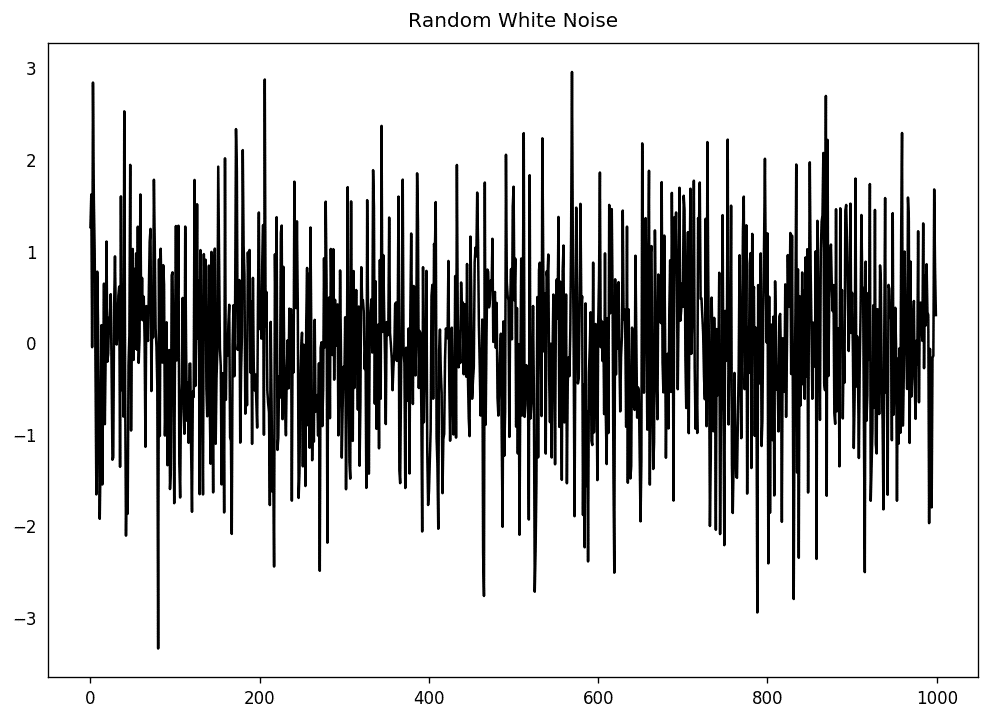Random White Noise

## 12. How to detrend a time series?

Detrending a time series is to remove the trend component from a time series. But how to extract the trend? There are multiple approaches.

1. Subtract the line of best fit from the time series. The line of best fit may be obtained from a linear regression model with the time steps as the predictor. For more complex trends, you may want to use quadratic terms (x^2) in the model.

2. Subtract the trend component obtained from time series decomposition we saw earlier.

3. Subtract the mean

4. Apply a filter like Baxter-King filter(statsmodels.tsa.filters.bkfilter) or the Hodrick-Prescott Filter (statsmodels.tsa.filters.hpfilter) to remove the moving average trend lines or the cyclical components.

Let’s implement the first two methods.

# Using scipy: Subtract the line of best fit
from scipy import signal
df = pd.read_csv('https://raw.githubusercontent.com/selva86/datasets/master/a10.csv', parse_dates=['date'])
detrended = signal.detrend(df.value.values)
plt.plot(detrended)
plt.title('Drug Sales detrended by subtracting the least squares fit', fontsize=16)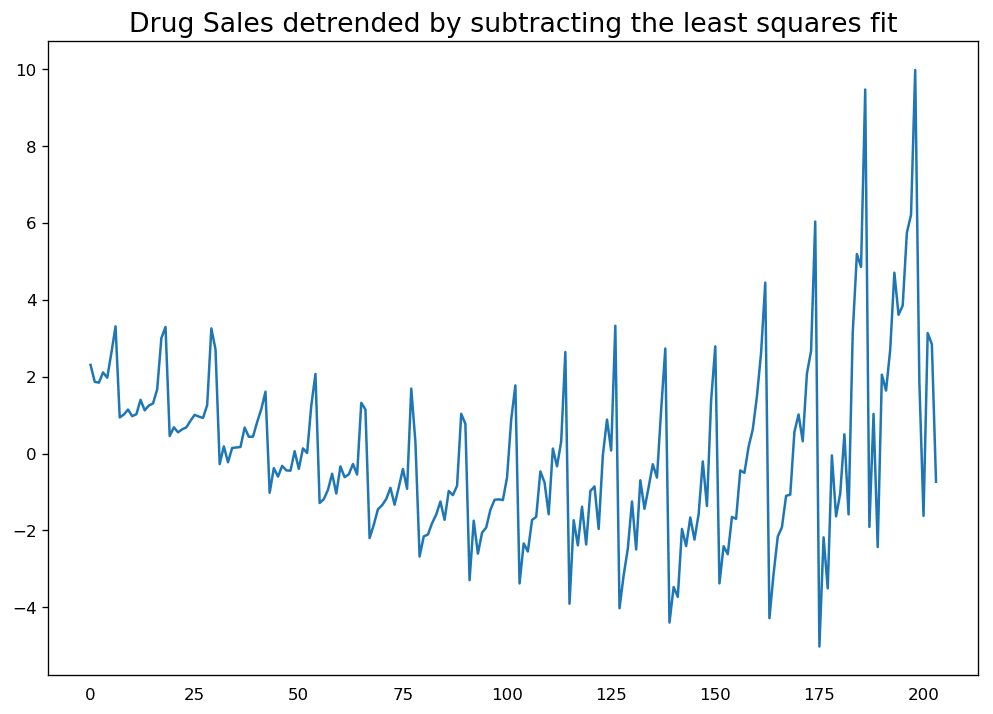Detrend A TimeSeries By Subtracting LeastSquaresFit
# Using statmodels: Subtracting the Trend Component.
from statsmodels.tsa.seasonal import seasonal_decompose
df = pd.read_csv('https://raw.githubusercontent.com/selva86/datasets/master/a10.csv', parse_dates=['date'], index_col='date')
result_mul = seasonal_decompose(df['value'], model='multiplicative', extrapolate_trend='freq')
detrended = df.value.values - result_mul.trend
plt.plot(detrended)
plt.title('Drug Sales detrended by subtracting the trend component', fontsize=16)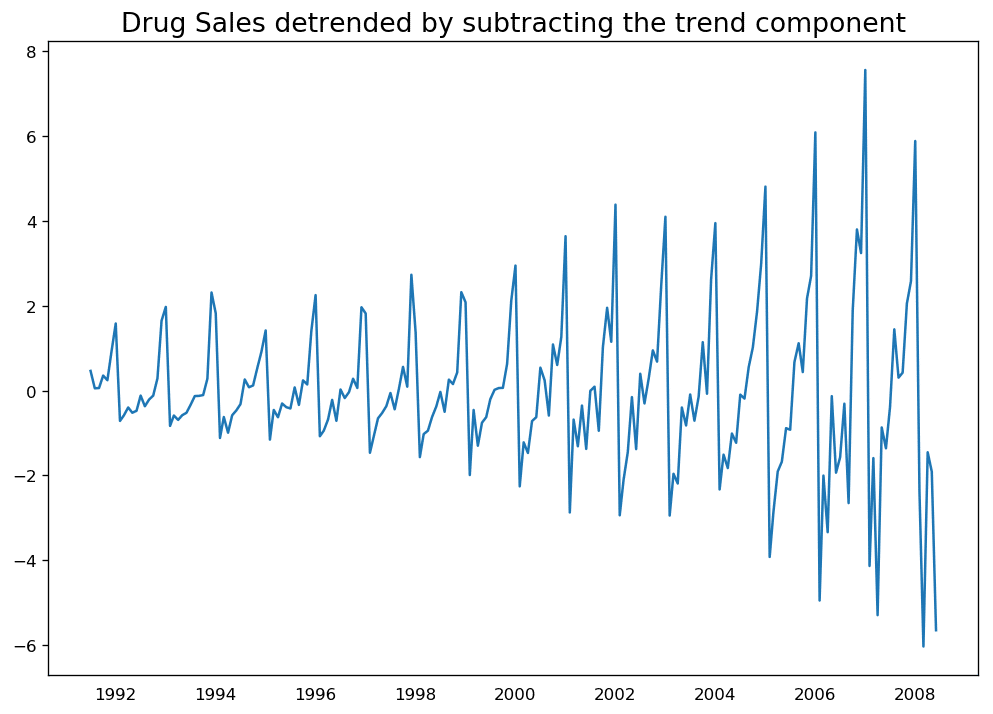Detrend By Subtracting Trend Component

## 13. How to deseasonalize a time series?

There are multiple approaches to deseasonalize a time series as well. Below are a few:

- 1. Take a moving average with length as the seasonal window. This will smoothen in series in the process.

- 2. Seasonal difference the series (subtract the value of previous season from the current value)

- 3. Divide the series by the seasonal index obtained from STL decomposition


If dividing by the seasonal index does not work well, try taking a log of the series and then do the deseasonalizing. You can later restore to the original scale by taking an exponential.

# Subtracting the Trend Component.
df = pd.read_csv('https://raw.githubusercontent.com/selva86/datasets/master/a10.csv', parse_dates=['date'], index_col='date')

# Time Series Decomposition
result_mul = seasonal_decompose(df['value'], model='multiplicative', extrapolate_trend='freq')

# Deseasonalize
deseasonalized = df.value.values / result_mul.seasonal

# Plot
plt.plot(deseasonalized)
plt.title('Drug Sales Deseasonalized', fontsize=16)
plt.plot()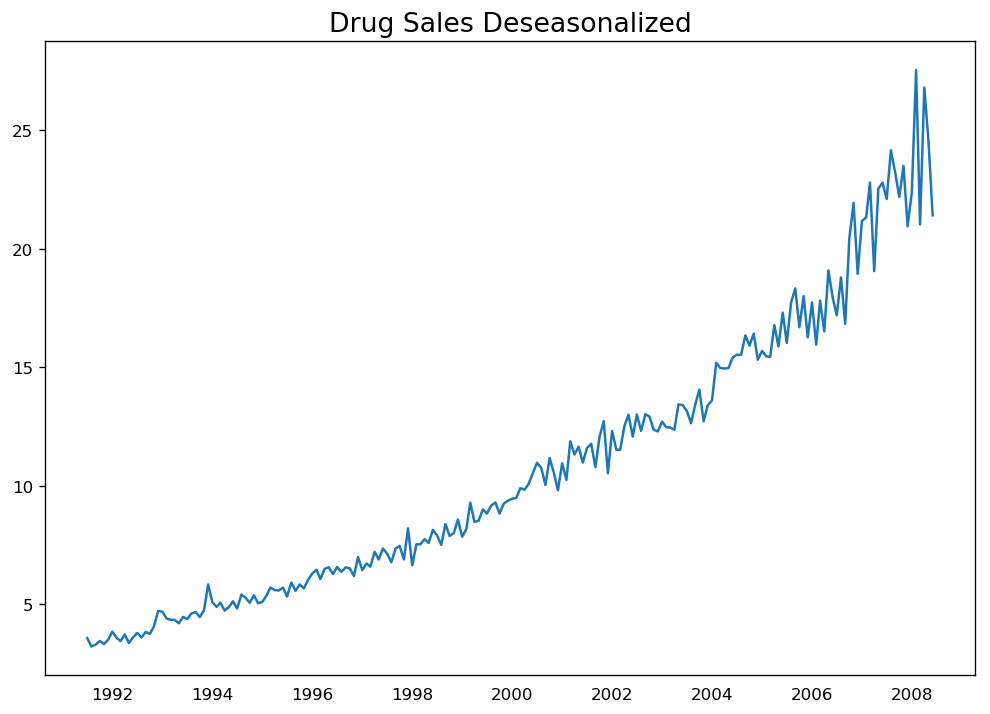Deseasonalize Time Series

## 14. How to test for seasonality of a time series?

The common way is to plot the series and check for repeatable patterns in fixed time intervals. So, the types of seasonality is determined by the clock or the calendar:

1. Hour of day
2. Day of month
3. Weekly
4. Monthly
5. Yearly

However, if you want a more definitive inspection of the seasonality, use the Autocorrelation Function (ACF) plot. More on the ACF in the upcoming sections. But when there is a strong seasonal pattern, the ACF plot usually reveals definitive repeated spikes at the multiples of the seasonal window.

For example, the drug sales time series is a monthly series with patterns repeating every year. So, you can see spikes at 12th, 24th, 36th.. lines.

I must caution you that in real word datasets such strong patterns is hardly noticed and can get distorted by any noise, so you need a careful eye to capture these patterns.

from pandas.plotting import autocorrelation_plot
df = pd.read_csv('https://raw.githubusercontent.com/selva86/datasets/master/a10.csv')

# Draw Plot
plt.rcParams.update({'figure.figsize':(9,5), 'figure.dpi':120})
autocorrelation_plot(df.value.tolist())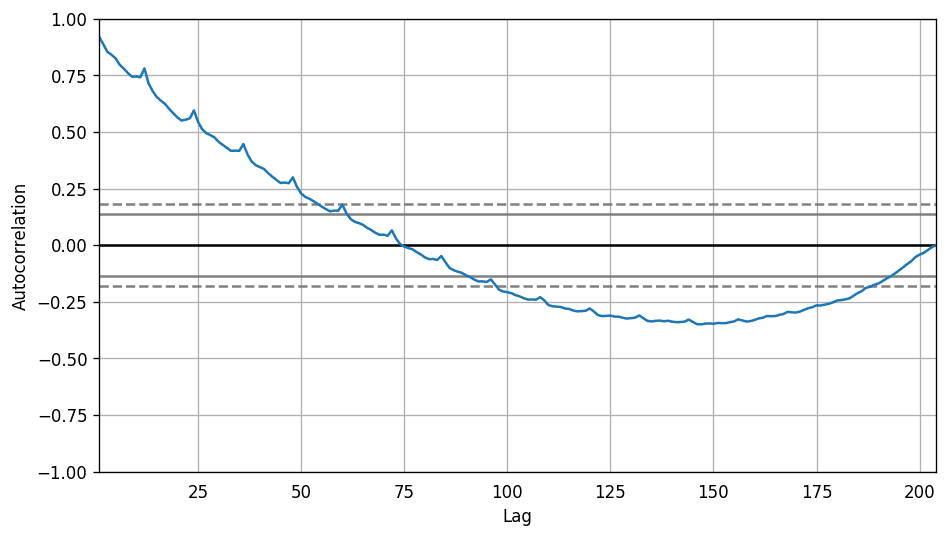Autocorrelation Plot

Alternately, if you want a statistical test, the CHTest can determine if seasonal differencing is required to stationarize the series.

## 15. How to treat missing values in a time series?

Sometimes, your time series will have missing dates/times. That means, the data was not captured or was not available for those periods. It could so happen the measurement was zero on those days, in which case, case you may fill up those periods with zero.

Secondly, when it comes to time series, you should typically NOT replace missing values with the mean of the series, especially if the series is not stationary. What you could do instead for a quick and dirty workaround is to forward-fill the previous value.

However, depending on the nature of the series, you want to try out multiple approaches before concluding. Some effective alternatives to imputation are:

• Backward Fill
• Linear Interpolation
• Quadratic interpolation
• Mean of nearest neighbors
• Mean of seasonal couterparts

To measure the imputation performance, I manually introduce missing values to the time series, impute it with above approaches and then measure the mean squared error of the imputed against the actual values.

# # Generate dataset
from scipy.interpolate import interp1d
from sklearn.metrics import mean_squared_error
df_orig = pd.read_csv('https://raw.githubusercontent.com/selva86/datasets/master/a10.csv', parse_dates=['date'], index_col='date').head(100)
df = pd.read_csv('datasets/a10_missings.csv', parse_dates=['date'], index_col='date')

fig, axes = plt.subplots(7, 1, sharex=True, figsize=(10, 12))
plt.rcParams.update({'xtick.bottom' : False})

## 1. Actual -------------------------------
df_orig.plot(title='Actual', ax=axes, label='Actual', color='red', style=".-")
df.plot(title='Actual', ax=axes, label='Actual', color='green', style=".-")
axes.legend(["Missing Data", "Available Data"])

## 2. Forward Fill --------------------------
df_ffill = df.ffill()
error = np.round(mean_squared_error(df_orig['value'], df_ffill['value']), 2)
df_ffill['value'].plot(title='Forward Fill (MSE: ' + str(error) +")", ax=axes, label='Forward Fill', style=".-")

## 3. Backward Fill -------------------------
df_bfill = df.bfill()
error = np.round(mean_squared_error(df_orig['value'], df_bfill['value']), 2)
df_bfill['value'].plot(title="Backward Fill (MSE: " + str(error) +")", ax=axes, label='Back Fill', color='firebrick', style=".-")

## 4. Linear Interpolation ------------------
df['rownum'] = np.arange(df.shape)
df_nona = df.dropna(subset = ['value'])
f = interp1d(df_nona['rownum'], df_nona['value'])
df['linear_fill'] = f(df['rownum'])
error = np.round(mean_squared_error(df_orig['value'], df['linear_fill']), 2)
df['linear_fill'].plot(title="Linear Fill (MSE: " + str(error) +")", ax=axes, label='Cubic Fill', color='brown', style=".-")

## 5. Cubic Interpolation --------------------
f2 = interp1d(df_nona['rownum'], df_nona['value'], kind='cubic')
df['cubic_fill'] = f2(df['rownum'])
error = np.round(mean_squared_error(df_orig['value'], df['cubic_fill']), 2)
df['cubic_fill'].plot(title="Cubic Fill (MSE: " + str(error) +")", ax=axes, label='Cubic Fill', color='red', style=".-")

# Interpolation References:
# https://docs.scipy.org/doc/scipy/reference/tutorial/interpolate.html
# https://docs.scipy.org/doc/scipy/reference/interpolate.html

## 6. Mean of 'n' Nearest Past Neighbors ------
def knn_mean(ts, n):
out = np.copy(ts)
for i, val in enumerate(ts):
if np.isnan(val):
n_by_2 = np.ceil(n/2)
lower = np.max([0, int(i-n_by_2)])
upper = np.min([len(ts)+1, int(i+n_by_2)])
ts_near = np.concatenate([ts[lower:i], ts[i:upper]])
out[i] = np.nanmean(ts_near)
return out

df['knn_mean'] = knn_mean(df.value.values, 8)
error = np.round(mean_squared_error(df_orig['value'], df['knn_mean']), 2)
df['knn_mean'].plot(title="KNN Mean (MSE: " + str(error) +")", ax=axes, label='KNN Mean', color='tomato', alpha=0.5, style=".-")

## 7. Seasonal Mean ----------------------------
def seasonal_mean(ts, n, lr=0.7):
"""
Compute the mean of corresponding seasonal periods
ts: 1D array-like of the time series
n: Seasonal window length of the time series
"""
out = np.copy(ts)
for i, val in enumerate(ts):
if np.isnan(val):
ts_seas = ts[i-1::-n]  # previous seasons only
if np.isnan(np.nanmean(ts_seas)):
ts_seas = np.concatenate([ts[i-1::-n], ts[i::n]])  # previous and forward
out[i] = np.nanmean(ts_seas) * lr
return out

df['seasonal_mean'] = seasonal_mean(df.value, n=12, lr=1.25)
error = np.round(mean_squared_error(df_orig['value'], df['seasonal_mean']), 2)
df['seasonal_mean'].plot(title="Seasonal Mean (MSE: " + str(error) +")", ax=axes, label='Seasonal Mean', color='blue', alpha=0.5, style=".-")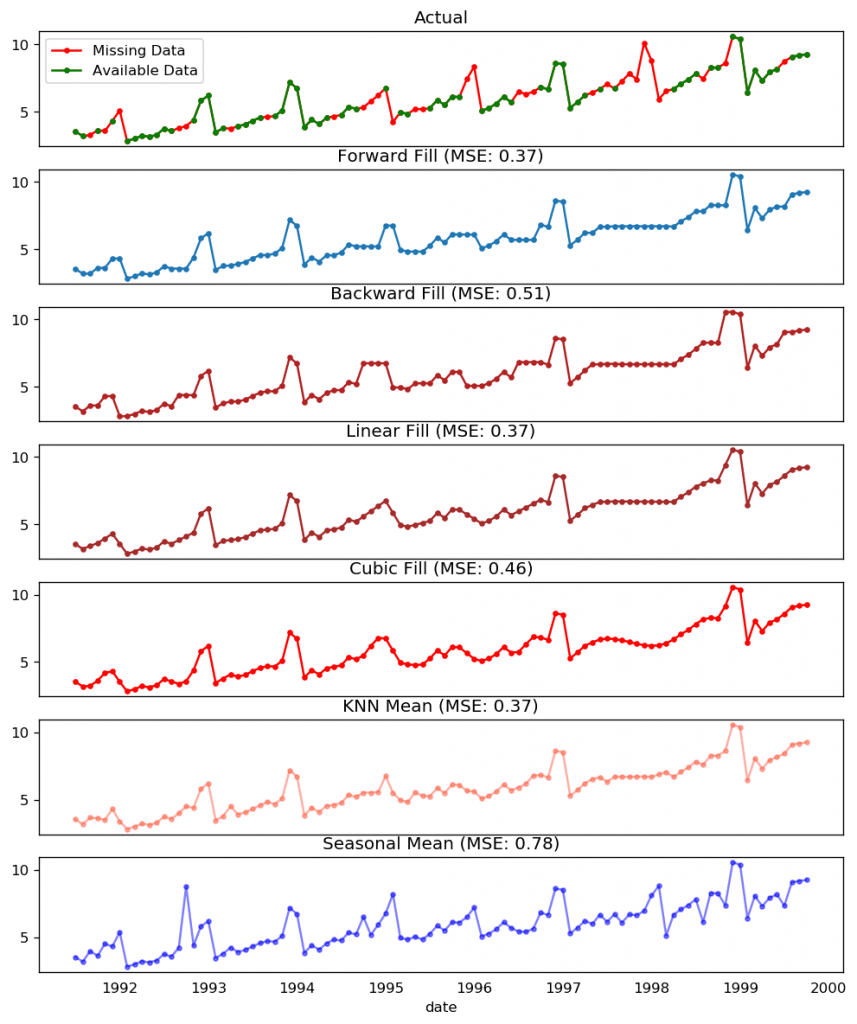Missing Value Treatments

You could also consider the following approaches depending on how accurate you want the imputations to be.

1. If you have explanatory variables use a prediction model like the random forest or k-Nearest Neighbors to predict it.
2. If you have enough past observations, forecast the missing values.
3. If you have enough future observations, backcast the missing values
4. Forecast of counterparts from previous cycles.

## 16. What is autocorrelation and partial autocorrelation functions?

Autocorrelation is simply the correlation of a series with its own lags. If a series is significantly autocorrelated, that means, the previous values of the series (lags) may be helpful in predicting the current value.

Partial Autocorrelation also conveys similar information but it conveys the pure correlation of a series and its lag, excluding the correlation contributions from the intermediate lags.

from statsmodels.tsa.stattools import acf, pacf
from statsmodels.graphics.tsaplots import plot_acf, plot_pacf

df = pd.read_csv('https://raw.githubusercontent.com/selva86/datasets/master/a10.csv')

# Calculate ACF and PACF upto 50 lags
# acf_50 = acf(df.value, nlags=50)
# pacf_50 = pacf(df.value, nlags=50)

# Draw Plot
fig, axes = plt.subplots(1,2,figsize=(16,3), dpi= 100)
plot_acf(df.value.tolist(), lags=50, ax=axes)
plot_pacf(df.value.tolist(), lags=50, ax=axes)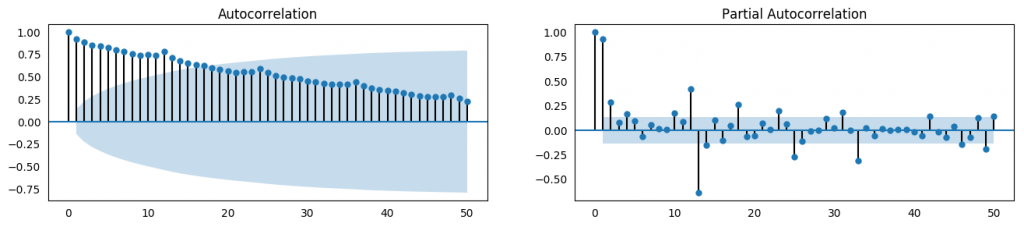ACF and PACF

## 17. How to compute partial autocorrelation function?

So how to compute partial autocorrelation?

The partial autocorrelation of lag (k) of a series is the coefficient of that lag in the autoregression equation of Y. The autoregressive equation of Y is nothing but the linear regression of Y with its own lags as predictors.

For Example, if Y_t is the current series and Y_t-1 is the lag 1 of Y, then the partial autocorrelation of lag 3 (Y_t-3) is the coefficient $\alpha_3$ of Y_t-3 in the following equation: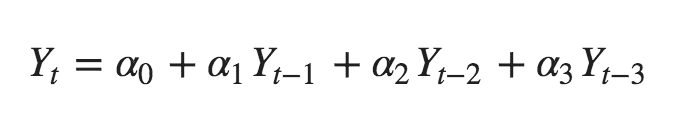Autoregression Equation

## 18. Lag Plots

A Lag plot is a scatter plot of a time series against a lag of itself. It is normally used to check for autocorrelation. If there is any pattern existing in the series like the one you see below, the series is autocorrelated. If there is no such pattern, the series is likely to be random white noise.

In below example on Sunspots area time series, the plots get more and more scattered as the n_lag increases.

from pandas.plotting import lag_plot
plt.rcParams.update({'ytick.left' : False, 'axes.titlepad':10})

# Import
ss = pd.read_csv('https://raw.githubusercontent.com/selva86/datasets/master/sunspotarea.csv')
a10 = pd.read_csv('https://raw.githubusercontent.com/selva86/datasets/master/a10.csv')

# Plot
fig, axes = plt.subplots(1, 4, figsize=(10,3), sharex=True, sharey=True, dpi=100)
for i, ax in enumerate(axes.flatten()[:4]):
lag_plot(ss.value, lag=i+1, ax=ax, c='firebrick')
ax.set_title('Lag ' + str(i+1))

fig.suptitle('Lag Plots of Sun Spots Area \n(Points get wide and scattered with increasing lag -> lesser correlation)\n', y=1.15)

fig, axes = plt.subplots(1, 4, figsize=(10,3), sharex=True, sharey=True, dpi=100)
for i, ax in enumerate(axes.flatten()[:4]):
lag_plot(a10.value, lag=i+1, ax=ax, c='firebrick')
ax.set_title('Lag ' + str(i+1))

fig.suptitle('Lag Plots of Drug Sales', y=1.05)
plt.show()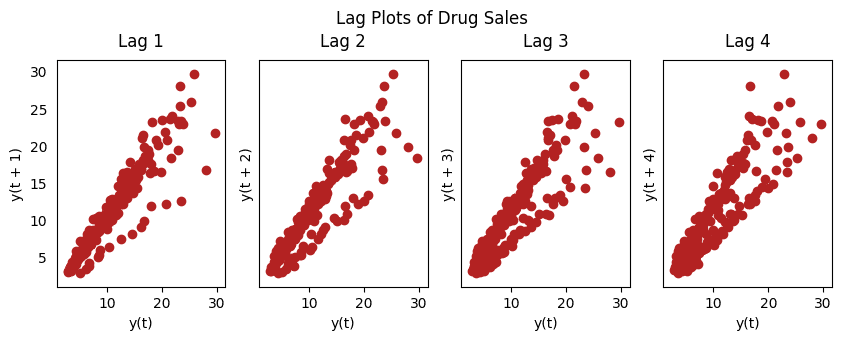Lagplots Drugsales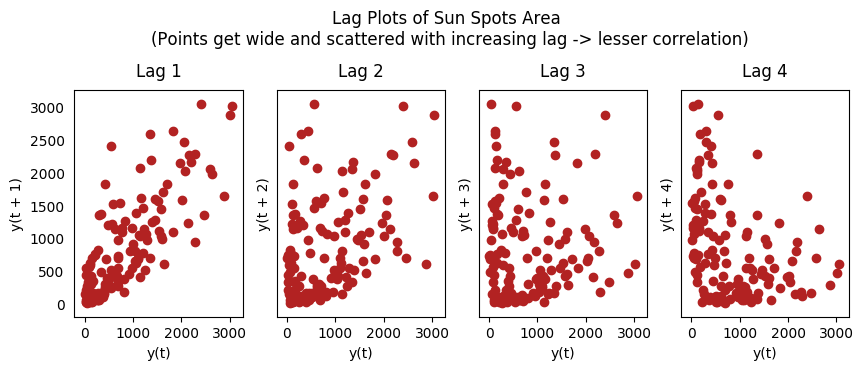Lagplots Sunspots

## 19. How to estimate the forecastability of a time series?

The more regular and repeatable patterns a time series has, the easier it is to forecast. The ‘Approximate Entropy’ can be used to quantify the regularity and unpredictability of fluctuations in a time series.

The higher the approximate entropy, the more difficult it is to forecast it.

Another better alternate is the ‘Sample Entropy’.

Sample Entropy is similar to approximate entropy but is more consistent in estimating the complexity even for smaller time series. For example, a random time series with fewer data points can have a lower ‘approximate entropy’ than a more ‘regular’ time series, whereas, a longer random time series will have a higher ‘approximate entropy’.

Sample Entropy handles this problem nicely. See the demonstration below.

# https://en.wikipedia.org/wiki/Approximate_entropy
ss = pd.read_csv('https://raw.githubusercontent.com/selva86/datasets/master/sunspotarea.csv')
a10 = pd.read_csv('https://raw.githubusercontent.com/selva86/datasets/master/a10.csv')
rand_small = np.random.randint(0, 100, size=36)
rand_big = np.random.randint(0, 100, size=136)

def ApEn(U, m, r):
"""Compute Aproximate entropy"""
def _maxdist(x_i, x_j):
return max([abs(ua - va) for ua, va in zip(x_i, x_j)])

def _phi(m):
x = [[U[j] for j in range(i, i + m - 1 + 1)] for i in range(N - m + 1)]
C = [len([1 for x_j in x if _maxdist(x_i, x_j) <= r]) / (N - m + 1.0) for x_i in x]
return (N - m + 1.0)**(-1) * sum(np.log(C))

N = len(U)
return abs(_phi(m+1) - _phi(m))

print(ApEn(ss.value, m=2, r=0.2*np.std(ss.value)))     # 0.651
print(ApEn(a10.value, m=2, r=0.2*np.std(a10.value)))   # 0.537
print(ApEn(rand_small, m=2, r=0.2*np.std(rand_small))) # 0.143
print(ApEn(rand_big, m=2, r=0.2*np.std(rand_big)))     # 0.716

0.6514704970333534
0.5374775224973489
0.0898376940798844
0.7369242960384561

# https://en.wikipedia.org/wiki/Sample_entropy
def SampEn(U, m, r):
"""Compute Sample entropy"""
def _maxdist(x_i, x_j):
return max([abs(ua - va) for ua, va in zip(x_i, x_j)])

def _phi(m):
x = [[U[j] for j in range(i, i + m - 1 + 1)] for i in range(N - m + 1)]
C = [len([1 for j in range(len(x)) if i != j and _maxdist(x[i], x[j]) <= r]) for i in range(len(x))]
return sum(C)

N = len(U)
return -np.log(_phi(m+1) / _phi(m))

print(SampEn(ss.value, m=2, r=0.2*np.std(ss.value)))      # 0.78
print(SampEn(a10.value, m=2, r=0.2*np.std(a10.value)))    # 0.41
print(SampEn(rand_small, m=2, r=0.2*np.std(rand_small)))  # 1.79
print(SampEn(rand_big, m=2, r=0.2*np.std(rand_big)))      # 2.42

0.7853311366380039
0.41887013457621214
inf
2.181224235989778

del sys.path


## 20. Why and How to smoothen a time series?

Smoothening of a time series may be useful in:

• Reducing the effect of noise in a signal get a fair approximation of the noise-filtered series.
• The smoothed version of series can be used as a feature to explain the original series itself.
• Visualize the underlying trend better

So how to smoothen a series? Let’s discuss the following methods:

1. Take a moving average
2. Do a LOESS smoothing (Localized Regression)
3. Do a LOWESS smoothing (Locally Weighted Regression)

Moving average is nothing but the average of a rolling window of defined width. But you must choose the window-width wisely, because, large window-size will over-smooth the series. For example, a window-size equal to the seasonal duration (ex: 12 for a month-wise series), will effectively nullify the seasonal effect.

LOESS, short for ‘LOcalized regrESSion’ fits multiple regressions in the local neighborhood of each point. It is implemented in the statsmodels package, where you can control the degree of smoothing using frac argument which specifies the percentage of data points nearby that should be considered to fit a regression model.

Download dataset: Elecequip.csv

from statsmodels.nonparametric.smoothers_lowess import lowess
plt.rcParams.update({'xtick.bottom' : False, 'axes.titlepad':5})

# Import
df_orig = pd.read_csv('datasets/elecequip.csv', parse_dates=['date'], index_col='date')

# 1. Moving Average
df_ma = df_orig.value.rolling(3, center=True, closed='both').mean()

# 2. Loess Smoothing (5% and 15%)
df_loess_5 = pd.DataFrame(lowess(df_orig.value, np.arange(len(df_orig.value)), frac=0.05)[:, 1], index=df_orig.index, columns=['value'])
df_loess_15 = pd.DataFrame(lowess(df_orig.value, np.arange(len(df_orig.value)), frac=0.15)[:, 1], index=df_orig.index, columns=['value'])

# Plot
fig, axes = plt.subplots(4,1, figsize=(7, 7), sharex=True, dpi=120)
df_orig['value'].plot(ax=axes, color='k', title='Original Series')
df_loess_5['value'].plot(ax=axes, title='Loess Smoothed 5%')
df_loess_15['value'].plot(ax=axes, title='Loess Smoothed 15%')
df_ma.plot(ax=axes, title='Moving Average (3)')
fig.suptitle('How to Smoothen a Time Series', y=0.95, fontsize=14)
plt.show()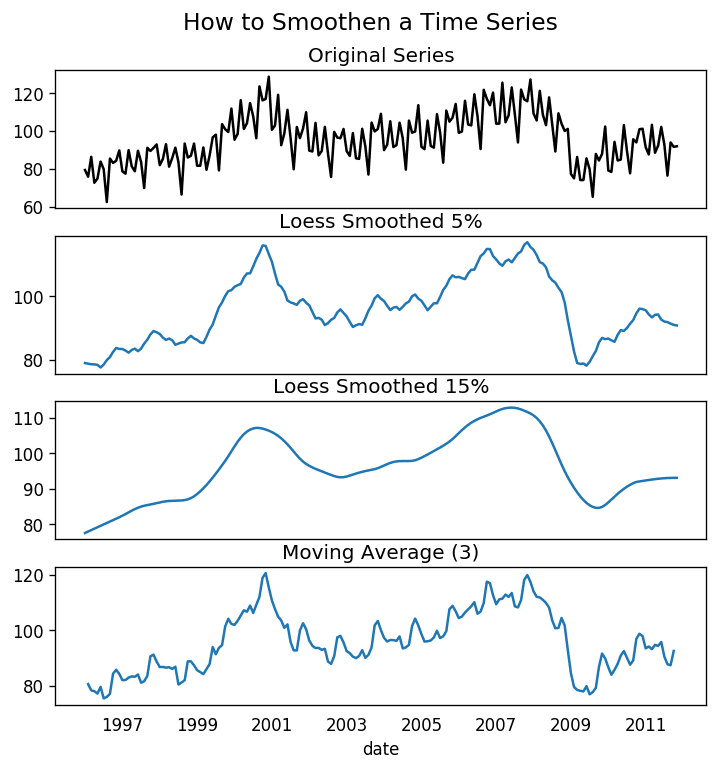Smoothen Timeseries

## How to use Granger Causality test to know if one time series is helpful in forecasting another?

Granger causality test is used to determine if one time series will be useful to forecast another.

How does Granger causality test work?

It is based on the idea that if X causes Y, then the forecast of Y based on previous values of Y AND the previous values of X should outperform the forecast of Y based on previous values of Y alone.

So, understand that Granger causality should not be used to test if a lag of Y causes Y. Instead, it is generally used on exogenous (not Y lag) variables only.

It is nicely implemented in the statsmodel package.

It accepts a 2D array with 2 columns as the main argument. The values are in the first column and the predictor (X) is in the second column.

The Null hypothesis is: the series in the second column, does not Granger cause the series in the first. If the P-Values are less than a significance level (0.05) then you reject the null hypothesis and conclude that the said lag of X is indeed useful.

The second argument maxlag says till how many lags of Y should be included in the test.

from statsmodels.tsa.stattools import grangercausalitytests
df = pd.read_csv('https://raw.githubusercontent.com/selva86/datasets/master/a10.csv', parse_dates=['date'])
df['month'] = df.date.dt.month
grangercausalitytests(df[['value', 'month']], maxlag=2)

Granger Causality
number of lags (no zero) 1
ssr based F test:         F=54.7797 , p=0.0000  , df_denom=200, df_num=1
ssr based chi2 test:   chi2=55.6014 , p=0.0000  , df=1
likelihood ratio test: chi2=49.1426 , p=0.0000  , df=1
parameter F test:         F=54.7797 , p=0.0000  , df_denom=200, df_num=1

Granger Causality
number of lags (no zero) 2
ssr based F test:         F=162.6989, p=0.0000  , df_denom=197, df_num=2
ssr based chi2 test:   chi2=333.6567, p=0.0000  , df=2
likelihood ratio test: chi2=196.9956, p=0.0000  , df=2
parameter F test:         F=162.6989, p=0.0000  , df_denom=197, df_num=2


In the above case, the P-Values are Zero for all tests. So the ‘month’ indeed can be used to forecast the Air Passengers.

## 22. What Next

That’s it for now. We started from the very basics and understood various characteristics of a time series. Once the analysis is done the next step is to begin forecasting.

In the next post, I will walk you through the in-depth process of building time series forecasting models using ARIMA. See you soon.## Download the Data Science Project Template

Reference project template for all your Data Science projects. Learn  how to load the data, get an overview of the data. Perform EDA, prepare data, build models & improve model performance.

## Similar Articles

Read More »

Read More »

### Logistic Regression – A Complete Tutorial With Examples in R

Read More »## Download the Source Code and FREE 17-page Resource Guide

Lorem Ipsum is simply dummy text of the printing and typesetting industry. Lorem Ipsum has been the industry’s standard dummy text ever since the Lorem Ipsum is simply dummy text of the printing and typesetting industry. Lorem Ipsum has been the industry’s standard dummy text ever since the